# Selenium元素定位方法总结

Selenium可以驱动浏览器完成各种操作，比如模拟点击等。要想操作一个元素，首先应该识别这个元素。人有各种的特征（属性），我们可以通过其特征找到人，如通过身份证号、姓名、家庭住址。同理，一个元素会有各种的特征（属性），我们可以通过这个属性找到这对象。

（一）什么是元素？

（二）查看元素信息

（一）根据id定位

（二）根据name定位

（三）通过class name定位

（四）根据tag定位

（七）根据XPath定位

2.7.1绝对路径

2.7.2相对路径

（八）通过css selector定位

2.8.1 id选择器

2.8.2 class选择器

2.8.3 标签选择器

2.8.4 属性选择器

2.8.5 直接在浏览器复制

（九）定位方法的使用

2.9.1 定位方式选择

2.9.2 find_element和find_elements方法

# 一、元素的概念

## （二）查看元素信息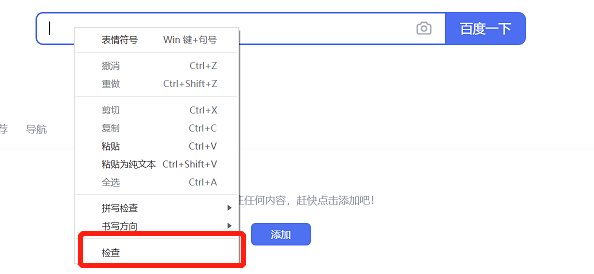image-20220511102134911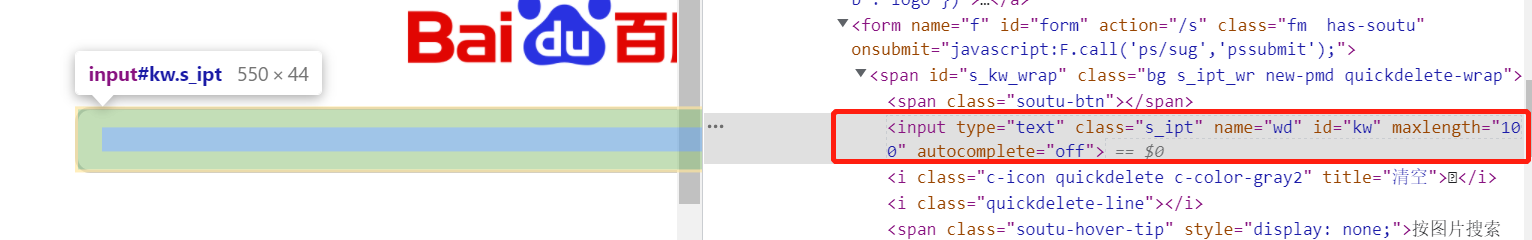image-20220510192728208

# 二、元素定位方法

webdriver 提供了一系列的对象定位方法，常用的有以下8种：

find_element_by_id find_elements_by_id 通过元素id定位
find_element_by_name find_elements_by_name 通过元素name定位
find_element_by_xpath find_elements_by_xpath 通过xpath表达式定位
find_element_by_tag_name find_elements_by_tag_name 通过标签定位
find_element_by_class_name find_elements_by_class_name 通过类名进行定位
find_element_by_css_selector find_elements_by_css_selector 通过css选择器进行定位

``````from selenium import webdriver

web = webdriver.Chrome()
web.get(r'https://www.baidu.com')
``````

## （一）根据id定位

`HTML`当中，id属性是唯一标识一个元素的属性，因此在`selenium`当中，通过id来进行元素的定位也作为首选。image-20220510192728208

``````<input type="text" class="s_ipt" name="wd" id="kw" maxlength="100" autocomplete="off">
``````

``````element = web.find_element_by_id("kw")
``````

## （二）根据name定位

`HTML`当中，name属性和id属性的功能基本相同，只是name属性并不是唯一的，如果遇到没有id标签的时候，我们可以考虑通过name标签来进行定位。

``````<input type="text" class="s_ipt" name="wd" id="kw" maxlength="100" autocomplete="off">
``````

``````element = web.find_element_by_name("wd")
``````

## （三）通过class name定位

``````<input type="text" class="s_ipt" name="wd" id="kw" maxlength="100" autocomplete="off">
``````

``````element = web.find_element_by_class_name('s_ipt')
``````

## （四）根据tag定位

`HTML`是通过`tag`来定义一类功能的，比如`input`是输入，`table`是表格，`tbody`是表格主体等。每个元素其实就是一个`tag`，由于一个`tag`用来定义一类功能，一个网页往往有很多同类`tag`，所以很难通过`tag`去区分不同的元素。image-20220510193305826

``````<input type="text" class="s_ipt" name="wd" id="kw" maxlength="100" autocomplete="off">
``````

``````element = web.find_element_by_tag_name('input')
``````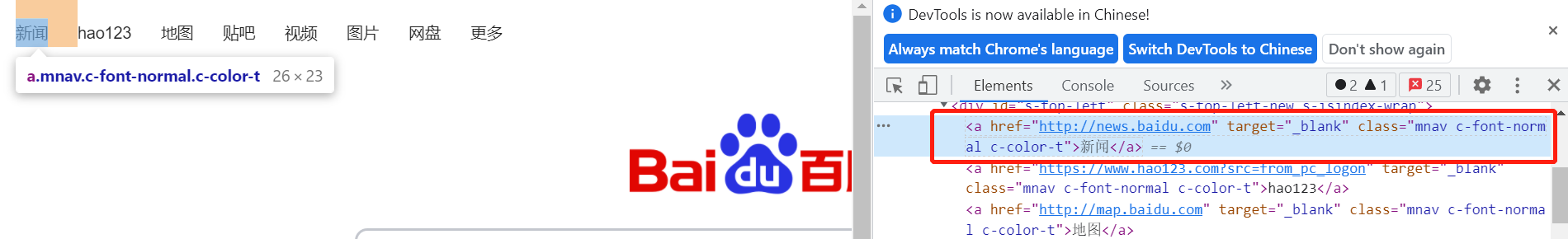image-20220510195131010

``````<a href="http://news.baidu.com" target="_blank" class="mnav c-font-normal c-color-t">新闻</a>
``````

``````element = web.find_element_by_link_text('新闻')
``````

``````<a href="http://news.baidu.com" target="_blank" class="mnav c-font-normal c-color-t">新闻</a>
``````

``````element = web.find_element_by_partial_link_text('闻')
``````

## （七）根据XPath定位

`Xpath`是一种在`XML``HTML`文档中查找信息的语言，通过`Xpath`路径来定位元素的时候也是分绝对路径和相对路径。

### 2.7.1绝对路径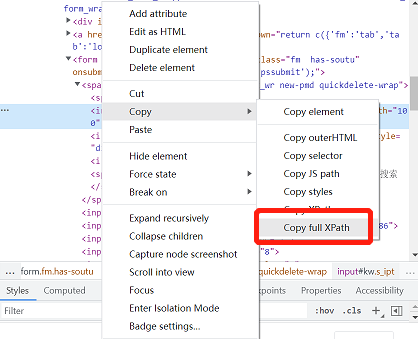image-20220510211910536

xpath定位表达式：

``````/html/body/div/div/div/div/div/form/span/input
``````

Python通过xpath定位语句：

``````element = web.find_element_by_xpath('/html/body/div/div/div/div/div/form/span/input')
``````

### 2.7.2相对路径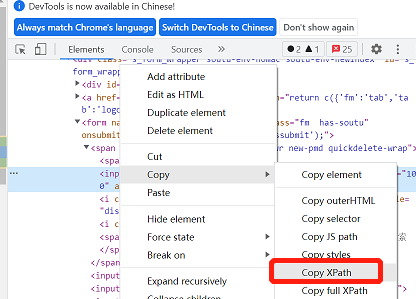image-20220510211834245

xpath定位表达式：

``````//*[@id="kw"]
``````

Python通过xpath定位语句：

``````element = web.find_element_by_xpath('//*[@id="kw"]')
``````

## （八）通过css selector定位

`Selenium`官网当中是更加推荐`Css Selector()`方法来进行页面元素的定位的，`Css`定位可以通过id选择器、class选择器、标签选择器和属性选择器。

### 2.8.1 id选择器

``````<input type="text" class="s_ipt" name="wd" id="kw" maxlength="100" autocomplete="off">
``````

``````element = web.find_element_by_css_selector("#kw")
``````

### 2.8.2 class选择器

``````<input type="text" class="s_ipt" name="wd" id="kw" maxlength="100" autocomplete="off">
``````

``````element = web.find_element_by_css_selector(".s_ipt")
``````

### 2.8.3 标签选择器

``````<input type="text" class="s_ipt" name="wd" id="kw" maxlength="100" autocomplete="off">
``````

``````element = web.find_element_by_css_selector("input")
``````

### 2.8.4 属性选择器

``````<input type="text" class="s_ipt" name="wd" id="kw" maxlength="100" autocomplete="off">
``````

``````element = web.find_element_by_css_selector('[id="kw"]')
element = web.find_element_by_css_selector('input[id="kw"]')
``````

2.8.4.1 定位带空格的复合class属性

``````class="s-top-left-new s-isindex-wrap"
``````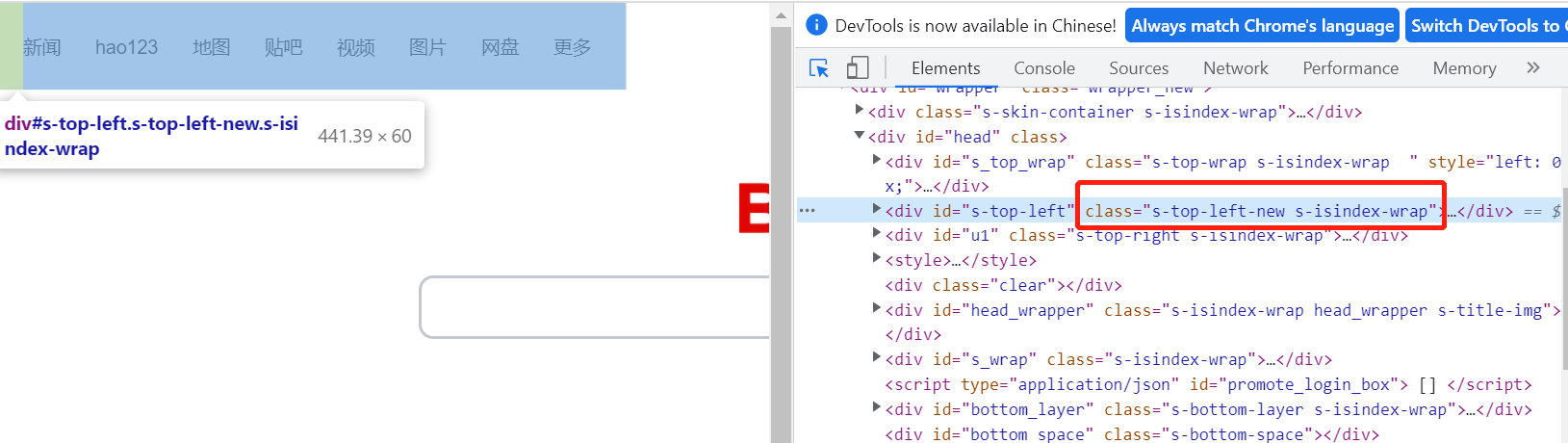image-20220511115348420

class属性带空格，如果直接通过class属性定位是会报错的，需要通过css selector按class属性定位。

``````element=web.find_element_by_class_name('s-top-left-new s-isindex-wrap')
``````

``````no such element: Unable to locate element: {"method":"css selector","selector":".s-top-left-new s-isindex-wrap"}
(Session info: chrome=99.0.4844.51)
``````

``````element=web.find_element_by_css_selector('[class="s-top-left-new s-isindex-wrap"]')
``````

### 2.8.5 直接在浏览器复制

css selector可以直接在浏览器中复制，鼠标单机右键-Copy-Copy selector即可获取。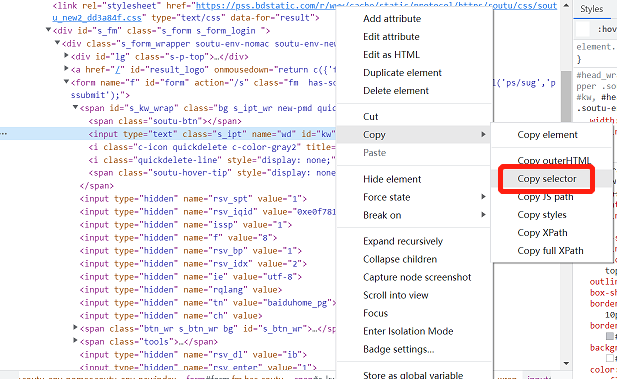image-20220511102825357

## （九）定位方法的使用

### 2.9.1 定位方式选择

• 当页面元素有id属性时，尽量用id来定位;

• 当要定位一组元素相同元素时，可以考虑用tag name或class name;

• css selector定位速度比较快，效率高。

• 一般id>name>css>XPath

### 2.9.2 find_element和find_elements方法

find_element_by_xx find_elements_by_xx

# 三、元素定位的另一种写法

`````` from selenium.webdriver.common.by import By
``````

``````from selenium.webdriver.common.by import By
element = web.find_element(By.ID,'kw')
element = web.find_element(By.NAME,'wd')
element = web.find_element(By.CLASS_NAME,'s_ipt')
element = web.find_element(By.TAG_NAME,'input')
element = web.find_element(By.XPATH,'//*[@id="kw"]')
element = web.find_element(By.CSS_SELECTOR,'#kw')
element = web.find_element(By.CSS_SELECTOR,'[id="kw"]')
element = web.find_element(By.CSS_SELECTOR,'input[id="kw"]')
``````

``````DeprecationWarning: find_element_by_* commands are deprecated. Please use find_element()
``````

``````pip install selenium==3.3.0
``````